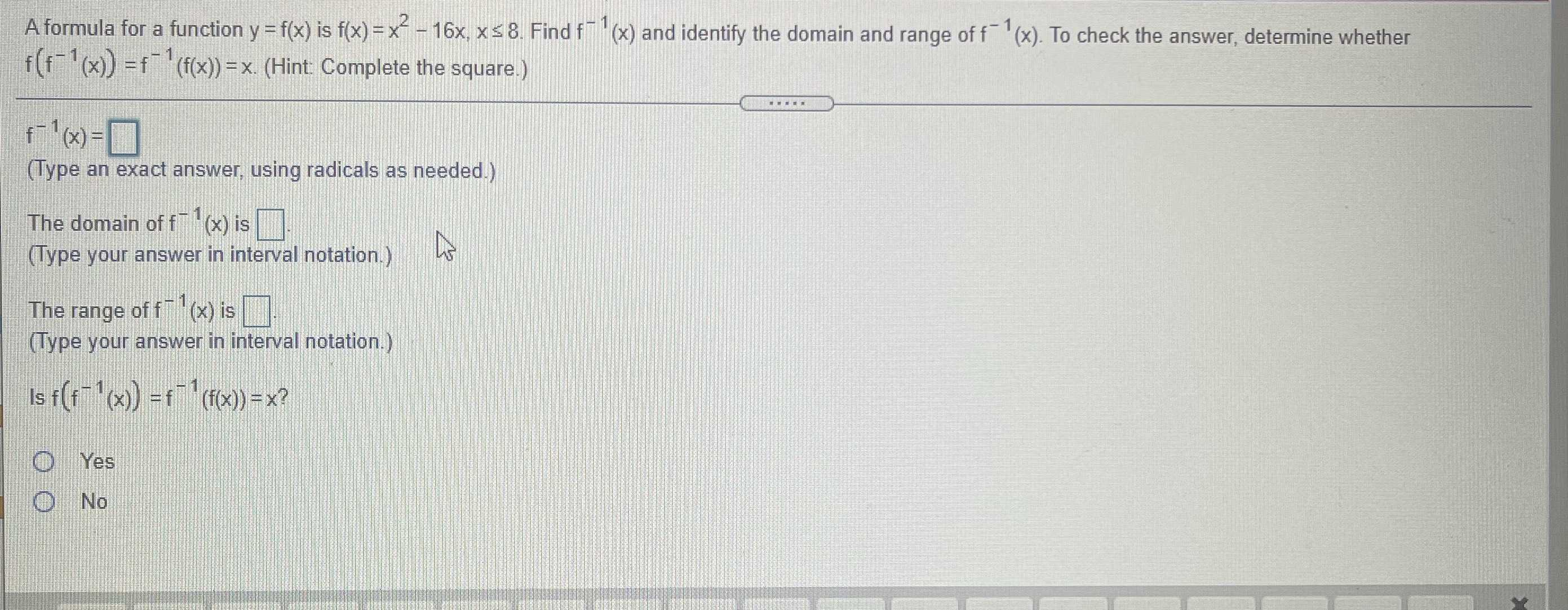### Still have math questions?

Algebra
QuestionA formula for a function $$y = f ( x )$$ is $$f ( x ) = x ^ { 2 } - 16 x , x \leq 8$$ . Find $$f ^ { - 1 } ( x )$$ and identify the domain and range of $$f ^ { - 1 } ( x )$$ . To check the answer, determine whether

$$f ( f ^ { - 1 } ( x ) ) = f ^ { - 1 } ( f ( x ) ) = x$$ . (Hint: Complete the square.) $$f ^ { - 1 } ( x ) = \square$$

(Type an exact answer, using radicals as needed.) The domain of $$f ^ { - 1 } ( x )$$ is $$\square$$

(Type your answer in interval notation.) The range of $$f ^ { - 1 } ( x )$$ is $$\square$$ . (Type your answer in interval notation.) Is $$f ( f ^ { - 1 } ( x ) ) = f ^ { - 1 } ( f ( x ) ) = x ?$$

Yes No

$$f^{- 1} (x)= 8- \sqrt{x+ 64}$$
domain    $$(x\ge - 64)$$
range    : $$(- \infty ,+ 8]$$